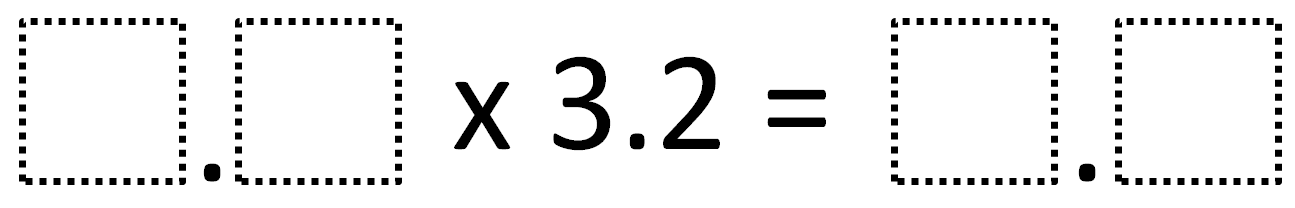Home > Grade 5 > Multiplying Decimals Given One

# Multiplying Decimals Given One

Directions: Using the digits 1 to 9 at most one time each, fill in the boxes to make a true statement.### Hint

What is the biggest the first number could be and still be less than 10?
What can we do to avoid having a product with non-zero digits in the hundredths place?

### Answer

The only answer is 1.5 x 3.2 = 4.8

Source: Robert Kaplinsky with help verifying the answer from Marcia and Rick Casterline

## Multiplying Decimals to Make a Whole Number Product

Directions: Using the digits 1 to 9 at most one time each, fill in the …

### 4 comments

1.I only found two possible answers: 1.5 and 2.5. Assumed zero was unavailable and found that placing any other digit in the tenths place created two digits to the right of the decimal point.

2.2.5 * 3.2 = 8.0, so if “0” is not an option, 1.5 * 3.2 = 4.8 is the ONLY answer

•Robert Kaplinsky

Neat! Thank you Marcia and Rick for figuring that out.

3.The answer is 1.5 x 3.2=4.8